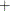# The ENTROPY Procedure (Experimental)

### RESTRICT Statement

• RESTRICT restriction1 < , restriction2 …>;

The RESTRICT statement is used to impose linear restrictions on the parameter estimates. You can specify any number of RESTRICT statements.

Each restriction is written as an optional name, followed by an expression, followed by an equality operator (=) or an inequality operator (<, >, <=, >=), followed by a second expression:

`<"name" > expression operator expression`

The optional "name" is a string used to identify the restriction in the printed output and in the OUTEST= data set. The operator can be =, <, >, <= , or >=. The operator and second expression are optional, as in the TEST statement, where they default to = 0.

Restriction expressions can be composed of variable names, multiplication (), and addition () operators, and constants. Variable names in restriction expressions must be among the variables whose coefficients are estimated by the model. The restriction expressions must be a linear function of the variables.

The following is an example of the use of the RESTRICT statement:

```proc entropy data=one;
restrict y1.x1*2 <= x2 + y2.x1;
model y1 = x1 x2;
model y2 = x1 x3;
run;
```

This example illustrates the use of compound names, `y1.x1`, to specify coefficients of specific equations.